When forces F1, F2, F3 are acting on a particle of mass m such that F2 and F3 are mutually perpendicular, then the particle remains stationary. If the force F1 is now removed then the acceleration of the particle is

(1) ${F}_{1}/m$

(2) ${F}_{2}{F}_{3}/m{F}_{1}$

(3) $\left({F}_{2}-{F}_{3}\right)/m$

(4) ${F}_{2}/m$

Concept Questions :-

Application of laws
High Yielding Test Series + Question Bank - NEET 2020

Difficulty Level:

Consider the following statement: When jumping from some height, you should bend your knees as you come to rest, instead of keeping your legs stiff. Which of the following relations can be useful in explaining the statement?

1. $\Delta \stackrel{\to }{{P}_{1}}=-\Delta \stackrel{\to }{{P}_{2}}$

2. $\Delta E=-\Delta \left(PE+KE\right)=0$

3. $\stackrel{\to }{F}\Delta t=m\Delta \stackrel{\to }{v}$

4. $\Delta \stackrel{\to }{x}\propto \Delta \stackrel{\to }{F}$

Concept Questions :-

Newton laws
High Yielding Test Series + Question Bank - NEET 2020

Difficulty Level:

A false balance has equal arms. An object weigh X when placed in one pan and Y when placed in other pan, then the weight W of the object is equal to

(1) $\sqrt{XY}$

(2) $\frac{X+Y}{2}$

(3) $\frac{{X}^{2}+{Y}^{2}}{2}$

(4) $\frac{2}{\sqrt{{X}^{2}+{Y}^{2}}}$

Concept Questions :-

Types of forces
High Yielding Test Series + Question Bank - NEET 2020

Difficulty Level:

In the arrangement shown in figure, the ends P and Q of an unstretchable string move downwards with uniform speed U. Pulleys A and B are fixed. Mass M moves upwards with a speed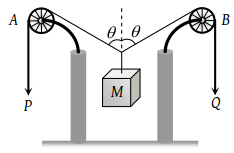1.

2.

3. $\frac{2U}{\mathrm{cos}\theta }$

4. $\frac{U}{\mathrm{cos}\theta }$

Concept Questions :-

String constraint
High Yielding Test Series + Question Bank - NEET 2020

Difficulty Level:

The pulleys and strings shown in the figure are smooth and of negligible mass. For the system to remain in equilibrium, the angle θ should be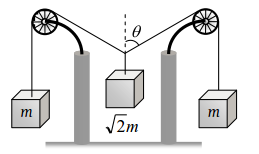(1) 0°

(2) 30°

(3) 45°

(4) 60°

High Yielding Test Series + Question Bank - NEET 2020

Difficulty Level:

A string of negligible mass going over a clamped pulley of mass m supports a block of mass M as shown in the figure. The force on the pulley by the clamp is given by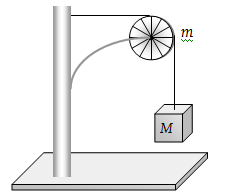(1) $\sqrt{2}Mg$

(2) $\sqrt{2}mg$

(3) $\sqrt{{\left(M+m\right)}^{2}+{m}^{2}}g$

(4) $\sqrt{{\left(M+m\right)}^{2}+{M}^{2}}g$

High Yielding Test Series + Question Bank - NEET 2020

Difficulty Level:

A pulley fixed to the ceilling carries a string with blocks of mass m and 3 m attached to its ends. The masses of string and pulley are negligible. When the system is released, its centre of mass moves with what acceleration

(1) 0

(2) g/4

(3) g/2

(4) –g/2

High Yielding Test Series + Question Bank - NEET 2020

Difficulty Level:

A solid sphere of mass 2 kg is resting inside a cube as shown in the figure. The cube is moving with a velocity $v=\left(5t\text{\hspace{0.17em}}\stackrel{^}{i}+2t\text{\hspace{0.17em}}\stackrel{^}{j}\right)m/s$. Here t is the time in second. All surface are smooth. The sphere is at rest with respect to the cube. What is the total force exerted by the sphere on the cube. (Take g = 10 m/s2)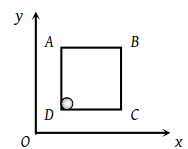1. $\sqrt{29}N$

2. 29 N

3. 26 N

4. $\sqrt{89}\text{\hspace{0.17em}}N$

Concept Questions :-

Types of forces
High Yielding Test Series + Question Bank - NEET 2020

Difficulty Level:

A block B is placed on block A. The mass of block B is less than the mass of block A. Friction exists between the blocks, whereas the ground on which the block A is placed is taken to be smooth. A horizontal force F, increasing linearly with time begins to act on B. The acceleration aA and aB of blocks A and B respectively are plotted against t. The correctly plotted graph is

 (1)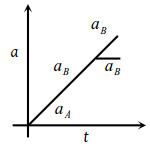(2)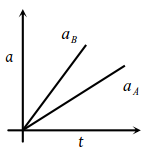(3)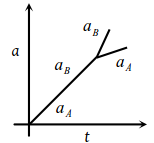(4)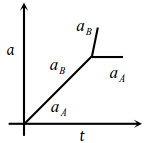Concept Questions :-

Friction
High Yielding Test Series + Question Bank - NEET 2020

Difficulty Level:

The force-time (Ft) curve of a particle executing linear motion is as shown in the figure. The momentum acquired by the particle in time interval from zero to 8 second will be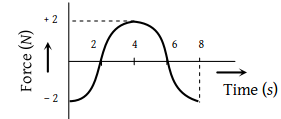(1) – 2 N-s

(2) + 4 N-s

(3) 6 N-s

(4) Zero

Concept Questions :-

Newton laws